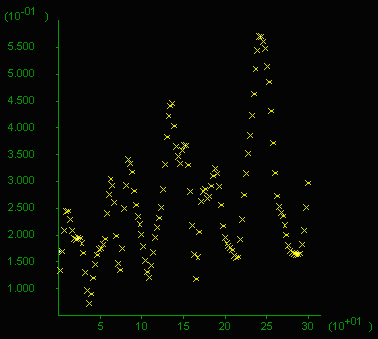# Shear Bands: Two Layer Models

### Roberto Weinberg, Monash University, AustraliaRyo Anma-san, University of Tsukuba, Japan

 Copyright 2005-2009 by Roberto Weinberg. All rights reserved. Unlimited permission to copy or use is hereby granted for non-profit driven enterprise subject to inclusion of this copyright notice and my World Wide Web URL: users.monash.edu.au/~weinberg. We would very much appreciate an email stating how this material will be used. Thanks, RW.

This section builds on the study of shear bands, stress distribution and fluid flow in media with layers of different elastic moduli.

MODEL SET UP

The model is a horizontal plane at a fixed lithostatic pressure (p), fluid absent and is deformed in pure shear with a horizontal shortening axis. The bulk modulus, K, and shear modulus G are such that they yield a Poisson ratio n = 0.25 and l = G. Fluids in the pores are included in some calculations at different pore pressures, and a horizontal layer with different elastic moduli is added in some calculations.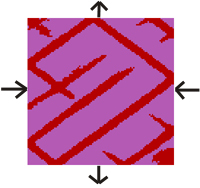Figure 1. Model 17: study of stress distribution in a layered system, the embedded layer in the middle has elastic moduli (Shear and Bulk Moduli) an order of magnitude higher than surroudings.

 A) State step 75000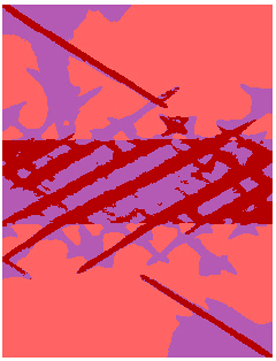B) Mean stress distributionC) Mean stress profile (zoom in a 400m profile)Figure 2. Model 18: study of stress distribution in a layered system, the embedded layer in the middle has elastic moduli (Shear and Bulk Moduli) an order of magnitude lower than surroudings.

 A) State step 75000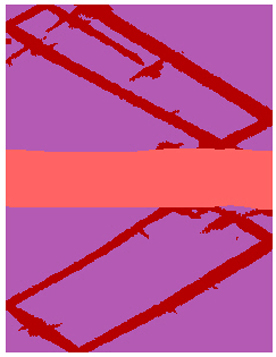B) Mean stress distribution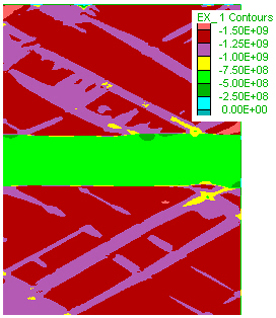C) Mean stress profile (zoom in a 400m profile)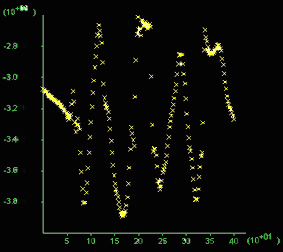Figure 3. Model 20. Like Figure 2 but pore fluids are initially at twice hydrostatic pressure. Step 55000. A) State. Much narrower and numerous shear bands develop compared to the case with no fluids (Fig. 1). Click on figure for movies. B) Is a zoom of a shear band
in A) and shows the fluid flow vectors indicating flow into the shear bands.
C) Pore pressure distribution showing a minimum in the center of the box.
D) Pore pressure profile showing a variation of 0.12 kbar across the area.

E) Mean stress distribution in a typical area approximately a quarter of the box area. F) Mean stress given in 10^8 Pa (=1 kbar) along a vertical section (x-axis in m), 200 m long through the middle of the box. Note the difference between highs and lows reaches 0.8 kbar and values oscilate around 4 kbar at this stage.
Click on figure to see a movie of the mean stress evolution.
G) Total volume flux vertical profile (in m3), x-axis in 100m.
H) Volume flux profile.

 A) State (movie)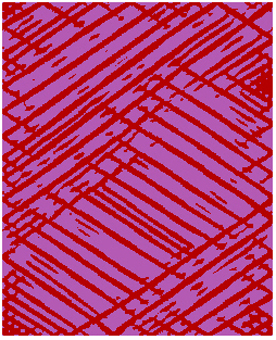B) Zoom of A) plus flow vectors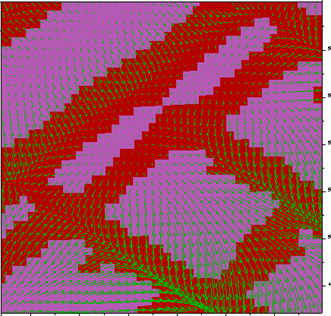C) Pore pressure (movie)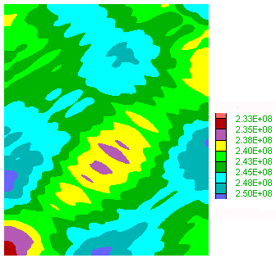D) Pore pressure profile (movie)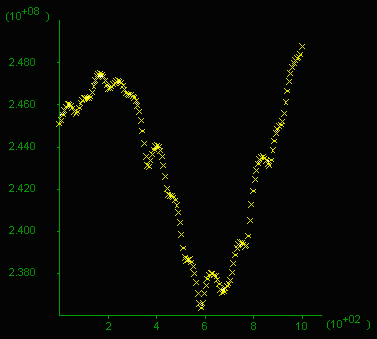E) Mean stess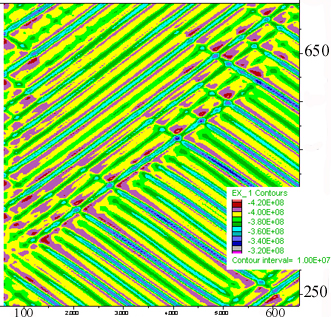F) Mean stress profile (movie)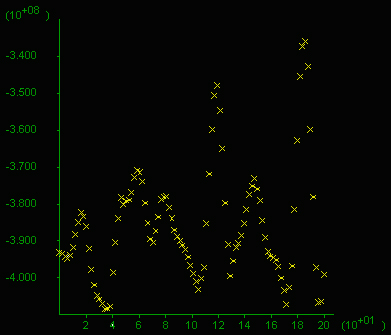G) Volume Flux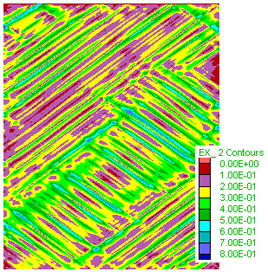H) Volume Flux Profile